# Filtering LightcurvesCIAO 4.11 Science Threads

## Overview

#### Synopsis:

It may be necessary to filter your data to remove periods of anomalous background levels, such as the flares seen in ACIS observations. The CIAO package includes the dmextract tool to calculate the lightcurve of a dataset (or region within a dataset), and the dmgti tool to create a GTI file given a table and a set of limits.

#### Purpose:

To analyze the light curve of the ACIS imaging background in order to clean the dataset of periods of anomalous background rates.

The algorithm used to detect flares is simple, so it may not be sufficient in all cases. If you intend to use the ACIS blank-sky background files, the cleaning described in this thread is not conservative enough. Instead, use the alternative method described in the Removing ACIS Background Flares thread.

Related Links:

Last Update: 30 Apr 2019 - Updated for contrib scripts 4.11.2 release; now using matplotlib for plotting.

## Get Started

Download the sample data: 1712 (ACIS-S, 3C 273); 3103 (Q1328+254)

`unix% download_chandra_obsid 1712,3103 evt2`

This thread uses the lc_sigma_clip routine from the lightcurves.py module. The deflare script may also be used to run lc_sigma_clip.

For this thread we shall restrict attention to the ACIS-S3 chip, and the 0.5 to 7 keV energy range:

```unix% punlearn dmcopy
unix% dmcopy "acisf01712N004_evt2.fits[energy=500:7000,ccd_id=7]" evt2_c7.fits
```

An image of the data is shown in Figure 1.

### Figure 1: ACIS-S3 observation of a field### Figure 1: ACIS-S3 observation of a field

ACIS-S3 observation in the 0.5 to 7 keV energy range.

## Remove bright/variable sources from the dataset

To avoid any background variations due to sources in the field we first filter out regions of high/variable emission from the dataset. There are a number of ways to create a list of regions that should be excluded - for instance you may wish to use the output from one of the source detection programs. In this thread we shall use visual estimation, as an example.

Using ds9, we chose several regions and saved them in CIAO format as exclude.reg, which looks like:

```unix% cat exclude.reg
# Region file format: CIAO version 1.0
rotbox(4200.3328,4137.9892,1129.5056,74.07019,24.22333)
circle(4076.5,4088.5,316)
circle(4296.5,5024.5,48)
```

The chosen regions are shown in Figure 2 (note that we ignored several obvious point sources in this example).

### Figure 2: "Source" regions### Figure 2: "Source" regions

For this example, the regions were chosen to contain most of the source emission.

## Create the lightcurve (dmextract)

We can now create a lightcurve of this background region using the CIAO dmextract tool. The best way to bin the lightcurve depends on the dataset and your scientific objectives; for this example we use a bin length of 200 seconds:

```unix% punlearn dmextract
unix% pset dmextract "evt2_c7.fits[exclude sky=region(exclude.reg)][bin time=::200]"
unix% pset dmextract outfile=lc_c7.fits
unix% pset dmextract opt=ltc1
unix% dmextract
Input event file  (evt2_c7.fits[exclude sky=region(exclude.reg)][bin time=::200]):
Enter output file name (lc_c7.fits):
```

The contents of the parameter file may be checked with plist dmextract.

## Analyze the lightcurve using lc_sigma_clip()

The lc_sigma_clip() routine provided by the lightcurves.py script performs an iterative sigma-clipping algorithm, removing those points that fall outside +/-3 sigma from the mean at each iteration until all data points are within +/-3 sigma (the number of sigma used to clip the data defaults to 3 but can be changed). This algorithm is robust but not perfect; it can easily "overclean" a noisy lightcurve and should not be used blindly. The routine can print out the limits used and can also create a GTI file that encodes these limits.

The deflare script provides a command-line interface to lc_sigma_clip. There is an example of using deflare in the running lc_sigma_clip via the deflare script section; see the ahelp file for more information.

The script must be loaded into ipython before it can be run (this step is only necessary once per session).

```>>> from lightcurves import *
```

If called with one argument - the name of the lightcurve to analyze - then the routine will use a 3-sigma clip, print out the resultant filter, and create a plot of the data (Figure 3):

```chips> lc_sigma_clip("lc_c7.fits")
Parameters used to clean the lightcurve are:
script version = February 2019
clipping       = symmetric
sigma          = 3
minlength      = 3
plot           = True
rateaxis       = y
color          = lime

Total number of bins in lightcurve   = 153
Max length of one bin                = 197.467 s
Num. bins with a smaller exp. time   = 2
Num. bins with exp. time = 0         = 13
Number of bins with a rate of 0 ct/s = 13

Rate filter:  -0.10548253792390716 <= count_rate < 1.023444242498134
Mean level of filtered lightcurve = 0.45898085228711338 ct/s

Warning: Default bin width of 0.01 count/s is too small as it produces
1151.5820249999977 bins.
Replacing with a width of 0.0115158 count/s
This may indicate that the lightcurve contains strong flares that
require manual filtering.

GTI limits calculated using a count-rate filter:
(count_rate>-0.10548253792390716 && count_rate<1.023444242498134)

The corresponding times are:
((time >= 77379470.949928001) && (time < 77399470.949928001)) ; 19.59 ksec, bin 1
((time >= 77404870.949928001) && (time < 77406670.949928001)) ; 1.78 ksec, bin 2

Exposure time of lightcurve = 27.45 ks
Filtered exposure time      = 21.36 ks
DTCOR value                 = 0.987337
```

### Figure 3: Plot from lc_sigma_clip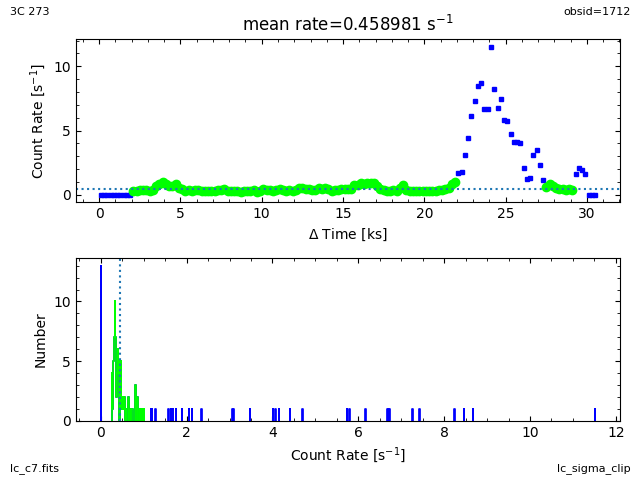### Figure 3: Plot from lc_sigma_clip

The top plot shows the light curve of lc_c7.fits, where the time axis is displayed on the x axis as a relative value (the first bin in the light curve is used as the 0 point). Points that are in green are those that passed the sigma-clipping and the horizontal dotted line shows the mean value of these points (the numerical value is given in the plot title).

The bottom plot shows a histogram of the count rate values; the green version again shows the data that passes the sigma-clipping algorithm.

Since there is a large flare, we re-run the analysis picking only those periods of the light curve with a "low" count rate. The limit used here is for demonstration purposes only; the value you should use depends on your science goals:

```chips> lc_sigma_clip("lc_c7.fits[count_rate<2]")
Parameters used to clean the lightcurve are:
script version = February 2019
clipping       = symmetric
sigma          = 3
minlength      = 3
plot           = True
rateaxis       = y
color          = lime

Total number of bins in lightcurve   = 130
Max length of one bin                = 197.467 s
Num. bins with a smaller exp. time   = 2
Num. bins with exp. time = 0         = 13
Number of bins with a rate of 0 ct/s = 13

Rate filter:  -0.10548253792390716 <= count_rate < 1.023444242498134
Mean level of filtered lightcurve = 0.45898085228711338 ct/s

GTI limits calculated using a count-rate filter:
(count_rate>-0.10548253792390716 && count_rate<1.023444242498134)

The corresponding times are:
((time >= 77379470.949928001) && (time < 77399470.949928001)) ; 19.59 ksec, bin 1
((time >= 77404870.949928001) && (time < 77406670.949928001)) ; 1.78 ksec, bin 2

Exposure time of lightcurve = 27.45 ks
Filtered exposure time      = 21.36 ks
DTCOR value                 = 0.987337
```

### Figure 4: Viewing the low count rate periods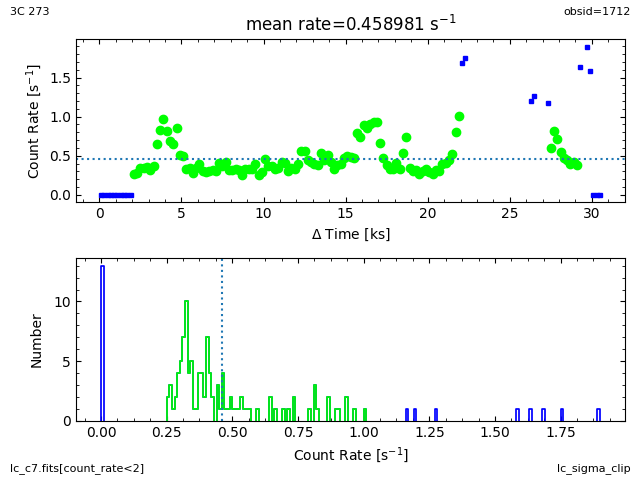### Figure 4: Viewing the low count rate periods

Note that this count-rate filter has been unable to remove all the flare periods. You can try lowering the maximum rate - e.g. to 0.7 - but you may find that for such strongly-flaring data that it is hard to select a "clean" set of times.

### Running lc_sigma_clip via the deflare script

The deflare script provides a command-line interface to lc_sigma_clip. Run deflare with method=sigma to choose the lc_sigma_clip cleaning method. To repeat the example from the previous section, the syntax would be:

```unix% deflare "lc_c7.fits[count_rate<2]" outfile=lc_c7.gti method=sigma
```

To create a plot of the filter, set plot=yes when running deflare.

For this part of the thread we switch to OBS_ID 3103. The setup to create the light curve file is similar to as before.

```unix% dmcopy "acisf03103N004_evt2.fits[energy=500:7000,ccd_id=7]" evt2_acis3103.fits
unix% cat exclude.reg
# Region file format: CIAO version 1.0
circle(4138.125,4036.75,9.4342425)
circle(4125.7479,4092.9526,3.2604091)
circle(4201.7609,3992.4783,3.5880036)
unix% dmextract "evt2_acis3103.fits[exclude sky=region(exclude.reg)][bin time=::500]" outfile=lc_acis3103_500s.fits dmextract opt=ltc1
```

By default, deflare filters out the times where the background count rate exceeds the ±3σ about the mean. These regions are indicated in red on the lightcurve that is displayed in Figure 5 for a lightcurve of ObsID 3103.

```unix% deflare acis3103_500s.lc outfile=3103.gti method="sigma" plot=yes \
save=deflare2.png

Parameters used to clean the lightcurve are:
script version = February 2019
clipping       = symmetric
sigma          = 3
minlength      = 3
outfile        = 3103.gti
plot           = True
rateaxis       = y
color          = lime
pattern        = solid
pattern color  = red

Total number of bins in lightcurve   = 83
Max length of one bin                = 453.474 s
Num. bins with a smaller exp. time   = 8
Num. bins with exp. time = 0         = 2
Number of bins with a rate of 0 ct/s = 2

Rate filter:  0.39413254668949571 <= count_rate < 0.61698993273852365
Mean level of filtered lightcurve = 0.50556123971400968 ct/s

GTI limits calculated using a count-rate filter:
(count_rate>0.39413254668949571 && count_rate<0.61698993273852365)

The corresponding times are:
((time >= 126705607.39342) && (time < 126707107.39342)) ; 0.97 ksec, bin 1
((time >= 126707607.39342) && (time < 126746107.39342)) ; 34.79 ksec, bin 2

Exposure time of lightcurve = 36.21 ks
Filtered exposure time      = 35.76 ks
DTCOR value                 = 0.906947

Creating GTI file
Created: 3103.gti
Light curve cleaned using the lc_sigma_clip routine.
Created: deflare2.png
Press ENTER to close the plot window and exit:
```

### Figure 5: Lightcurve clipped at 3σ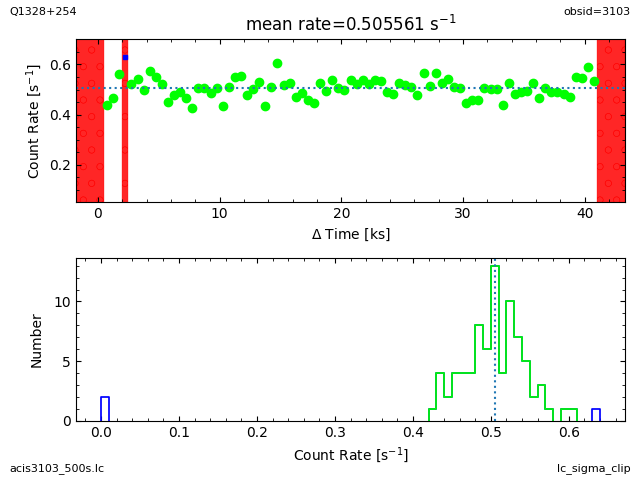### Figure 5: Lightcurve clipped at 3σ

Lightcurve filtered using lc_sigma_clip with the default values, including clipping any points outside ±3σ about the mean.

It is possible to filter the lightcurve more aggressively, by rejecting times with count rates that are close to the mean after each iteration. To reject times with count rates exceeding ±2σ about the mean (corresponding to a confidence level of ~90%), set the nsigma parameter:

```unix% deflare acis3103_500s.lc outfile=3103_1.6.gti method="sigma" \
plot=yes nsigma=2 save=deflare_03.png
Parameters used to clean the lightcurve are:
script version = February 2019
clipping       = symmetric
sigma          = 2
minlength      = 3
outfile        = 3103_1.6.gti
plot           = True
rateaxis       = y
color          = lime
pattern        = solid
pattern color  = red

Total number of bins in lightcurve   = 83
Max length of one bin                = 453.474 s
Num. bins with a smaller exp. time   = 8
Num. bins with exp. time = 0         = 2
Number of bins with a rate of 0 ct/s = 2

Rate filter:  0.45625311532574908 <= count_rate < 0.55778763136375964
Mean level of filtered lightcurve = 0.50702037334475436 ct/s

GTI limits calculated using a time filter:
((time >= 126707607.39342) && (time < 126709107.39342)) ; 1.36 ksec, bin 1
((time >= 126711107.39342) && (time < 126712607.39342)) ; 1.36 ksec, bin 2
((time >= 126713107.39342) && (time < 126715107.39342)) ; 1.81 ksec, bin 3
((time >= 126715607.39342) && (time < 126718607.39342)) ; 2.72 ksec, bin 4
((time >= 126720107.39342) && (time < 126722607.39342)) ; 2.27 ksec, bin 5
((time >= 126723107.39342) && (time < 126731607.39342)) ; 7.71 ksec, bin 6
((time >= 126733107.39342) && (time < 126735107.39342)) ; 1.81 ksec, bin 7
((time >= 126735607.39342) && (time < 126738107.39342)) ; 2.27 ksec, bin 8
((time >= 126738607.39342) && (time < 126745107.39342)) ; 5.89 ksec, bin 9

Exposure time of lightcurve = 36.21 ks
Filtered exposure time      = 27.21 ks
DTCOR value                 = 0.906947

Creating GTI file
Created: 3103_1.6.gti
Light curve cleaned using the lc_sigma_clip routine.
Created: deflare_03.png
Press ENTER to close the plot window and exit:
```

A larger number of time intervals have been rejected with the 2σ clipping, as shown in Figure 6.

### Figure 6: Lightcurve clipped at 2σ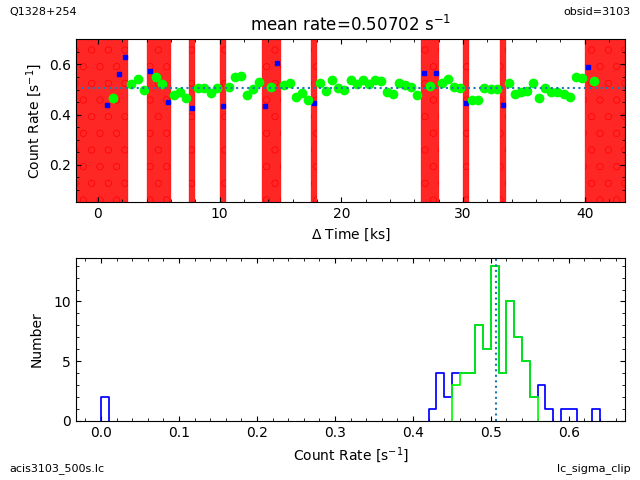### Figure 6: Lightcurve clipped at 2σ

The lightcurve is filtered by clipping any count rates outside of ±2σ from the mean. This is done by setting the deflare parameter nsigma=2, which is equivalent to setting the lc_sigma_clip parameter, sigma=2.

## Filtering the event file

The output time periods can be used to filter the event list. The lc_sigma_clip routine can create a GTI file directly, or you can use the limits it calculates to create the file yourself using the dmgti tool, or use them directly within a DM filter expression, as described below.

### 1. Using lc_sigma_clip

When the outfile parameter is set, lc_sigma_clip creates a GTI file:

```>>> from lightcurves import *
>>> lc_sigma_clip("lc_c7.fits[count_rate<2]", outfile="lc_c7.gti")
Parameters used to clean the lightcurve are:
script version = February 2019
clipping       = symmetric
sigma          = 3
minlength      = 3
outfile        = lc_c7.gti
plot           = True
rateaxis       = y
color          = lime
pattern        = solid
pattern color  = red

Total number of bins in lightcurve   = 130
Max length of one bin                = 197.467 s
Num. bins with a smaller exp. time   = 2
Num. bins with exp. time = 0         = 13
Number of bins with a rate of 0 ct/s = 13

Rate filter:  -0.10548253792390716 <= count_rate < 1.023444242498134
Mean level of filtered lightcurve = 0.45898085228711338 ct/s

GTI limits calculated using a count-rate filter:
(count_rate>-0.10548253792390716 && count_rate<1.023444242498134)

The corresponding times are:
((time >= 77379470.949928001) && (time < 77399470.949928001)) ; 19.59 ksec, bin 1
((time >= 77404870.949928001) && (time < 77406670.949928001)) ; 1.78 ksec, bin 2

Exposure time of lightcurve = 27.45 ks
Filtered exposure time      = 21.36 ks
DTCOR value                 = 0.987337

Creating GTI file
Created: lc_c7.gti
```

The regions filtered out are indicated by a solid red pattern as shown in Figure 7.

### Figure 7: Plot from lc_sigma_clip when outfile is set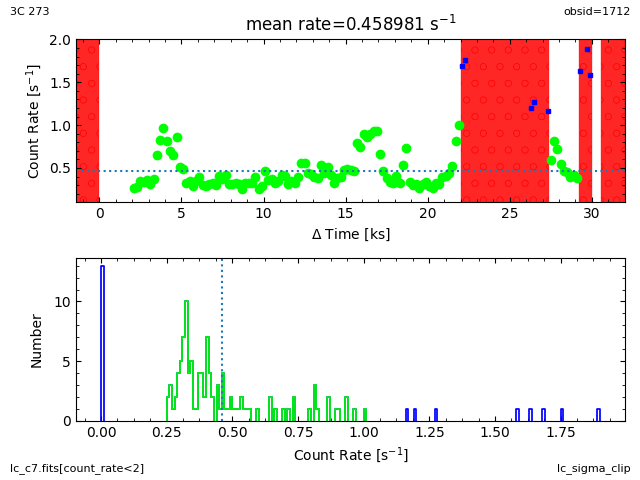### Figure 7: Plot from lc_sigma_clip when outfile is set

The top plot now indicates the regions excluded by the GTI file created by lc_sigma_clip - here lc_c7.gti - using a solid red pattern.

If you do not want a plot created then you can set the plot option to False; you may also want to set the verbose option to 0 to remove the screen output. For example:

```>>> lc_sigma_clip("lc_c7.fits", outfile="lc_c7.gti", plot=False, verbose=0)
```

See "ahelp lc_sigma_clip" for a full list of options.

The output file lc_c7.gti can then be used to filter an event list:

```unix% dmcopy "evt2_c7.fits[@lc_c7.gti]" evt2_c7_lc.fits
```

### 2. Using dmgti

The time limits can be used by dmgti to create a GTI file, as shown below (note that the individual ranges are combined by "||"):

```unix% punlearn dmgti
unix% pset dmgti infile=lc_c7.fits
unix% pset dmgti outfile=lc_c7.dmgti.gti
unix% pset dmgti \
userlimit="((time >= 77379470.949928) && (time < 77399470.949928))||((time >= 77404870.949928) && (time < 77406670.949928))"
unix% dmgti
Input MTL file (lc_c7.fits):
Output GTI file (lc_c7.dmgti.gti):
User defined limit string ((time >= 77379470.949928) && (time <
77399470.949928))||((time >= 77404870.949928) && (time <
77406670.949928))

```

The contents of the parameter file may be checked with plist dmgti.

The output of dmgti, here lc_c7.dmgti.gti, can then be used to filter an event list:

```unix% dmcopy "evt2_c7.fits[@lc_c7.dmgti.gti]" evt2_c7_dmgti.fits
```

### 3. Using a DM filter

Alternatively, you can use filter the event list directly using the column filtering capabilities of the CIAO Data Model:

```unix% dmcopy \
"evt2_c7.fits[time=77379470.949928:77399470.949928,77404870.949928:77406670.949928]" \
evt2_c7_dmcopy.fits
```

The final, filtered, exposure time can be found by looking at the EXPOSURE keyword of the filtered file:

```unix% dmkeypar evt2_c7_lc.fits EXPOSURE echo+
21362.821808294

unix% dmkeypar evt2_c7_dmgti.fits EXPOSURE echo+
21362.821808294

unix% dmkeypar evt2_c7_dmcopy.fits EXPOSURE echo+
21362.821808294
```

```
Parameters for /home/username/cxcds_param/dmextract.par

#--------------------------------------------------------------------
#
# DMEXTRACT -- extract columns or counts from an event list
#
#--------------------------------------------------------------------
infile = evt2_c7.fits[exclude sky=region(exclude.reg)][bin time=::200] Input event file
outfile = lc_c7.fits       Enter output file name
(bkg = )                Background region file or fixed background (counts/pixel/s) subtraction
(error = gaussian)        Method for error determination(poisson|gaussian|<variance file>)
(bkgerror = gaussian)        Method for background error determination(poisson|gaussian|<variance file>)
(bkgnorm = 1.0)             Background normalization
(exp = )                Exposure map image file
(bkgexp = )                Background exposure map image file
(sys_err = 0)               Fixed systematic error value for SYS_ERR keyword
(opt = ltc1)            Output file type: pha1
(defaults = \${ASCDS_CALIB}/cxo.mdb -> /soft/ciao/data/cxo.mdb) Instrument defaults file
(wmap = )                WMAP filter/binning (e.g. det=8 or default)
(clobber = no)              OK to overwrite existing output file(s)?
(verbose = 0)               Verbosity level
(mode = ql)
```

```
Parameters for /home/username/cxcds_param/dmgti.par

infile = lc_c7.fits       Input MTL file
outfile = lc_c7.dmgti.gti  Output GTI file
userlimit = ((time >= 77379470.949928) && (time < 77399470.949928))||((time >= 77404870.949928) && (time < 77406670.949928)) User defined limit string
(mtlfile = none)            Optional output smoothed/filtered MTL file
(lkupfile = none)            Lookup table defining which MTL columns to check against (NONE|none|<filename>)
(smooth = yes)             Smooth the input MTL data?
(clobber = no)              Clobber output file if it exists?
(verbose = 0)               Debug level
(mode = ql)
```

## History

 14 Dec 2004 updated for CIAO 3.2: path for script 08 Dec 2005 updated for CIAO 3.3: default value of dmextract error and bkgerror parameters is "gaussian" 01 Dec 2006 updated for CIAO 3.4: dmgti uses the value of the TIMEPIXR header keyword to adjust start and stop times (users may see a small shift in the time filter when compared to CIAO 3.3 because of this); kernel parameter removed from dmgti; ChIPS version 18 Jan 2008 updated for CIAO 4.0: analyze_ltcrv.sl v1.6 (plotting routines have been removed from the script; plotting will be replaced once it is updated for the new ChIPS syntax); filename and screen output updated for reprocessed data (version N004 event file); slsh version 0.8.2-0/S-Lang version 2.1.3 06 May 2008 changed energy filter to 0.5 to 7 keV (500:7000) 23 Jun 2008 updated image display to place figures inline with text 15 Dec 2008 updated for CIAO 4.1: analyze_ltcrv.sl has been replaced by lightcurves.sl and the routine analyze_ltcrv() by lc_sigma_clip(); added a Python version of the script; plotting capabilities have been restored; the routine can now generate a GTI file directly. 06 May 2009 check the version of the CIAO scripts package instead of the individual script 11 Feb 2010 updated for CIAO 4.2: added an example of filtering on count rate 05 Mar 2010 added a subsection on using deflare script; updated script tarfile version to 05 Mar 2010 13 Jan 2011 reviewed for CIAO 4.3: no changes 11 Jan 2012 reviewed for CIAO 4.4: added links to new Removing ACIS Background Flares thread 19 Jul 2012 fixed broken links 03 Dec 2012 Review for CIAO 4.5; updated file versions; 02 Dec 2013 Review for CIAO 4.6; no changes. 17 Dec 2014 Review for CIAO 4.7; no changes. 05 Jan 2017 Review for CIAO 4.9. Added info about obsid 3103 which is used part way through the thread. 30 Apr 2019 Updated for contrib scripts 4.11.2 release; now using matplotlib for plotting.